## Coordinate Geometry as a Tool for Proofs and Computations

### Coordinate Geometry as a Tool for Proofs and Computations## Coordinate Proofs

Writing proofs is an essential part of any high school geometry course. Consider, for instance, the triangle midsegment theorem, which states “A midsegment of a triangle, which is a line segment connecting the midpoints of two sides, is parallel to the third side and exactly half its length. For example, in the figure below,is the midsegment of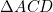. The usual “two-column” proof of this theorem goes as follows:Statement Reason , HypothesisSAS similarity. Corresponding angles in similar triangles are congruent. If a transversal intersecting and creates corresponding angles which are congruent, these segments are parallel. The ratios of corresponding sides of similar triangles are all equal. E is the midpoint of AD. Substitution (Q.E.D.)

The proof above did not actually involve any calculations, but instead took our hypothesis as the starting point and then followed a chain of logic involving various theorems in geometry until arriving at the conclusion. This kind of reasoning is new to most students and can be quite challenging (though they should still spend considerable time and effort to master this).

However, there is another way to prove this theorem which instead translates the problem completely into algebra. We begin by placing the triangle in the coordinate plane, assigning coordinates to the vertices  AC and D. Since we are free to choose how we position the triangle in the plane, for simplicity we place vertex A at the origin and align sidealong the x-axis. We can then prove this theorem by using the following facts from coordinate geometry:

1. The midpoint of a segment with endpoints and is given by
.
2. The length of a segment with endpoints and is given by
.
1. The slope of a segment with endpoints and is given by
.
2. Two segments are parallel if and only if their slopes are equal.The proof of the theorem is now easily carried out through straightforward calculations. Working out the coordinates of and using the midpoint formula,

Midpoint of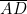:

Midpoint of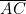:

We compute the slope ofto be

.

This is equal to the slope of, so these lines are parallel.

Computing now the length of, we find

,

which is exactly half the length of. (Q.E.D.)

Coordinate geometry also lends itself well to proofs involving quadrilaterals. For instance, a common problem is to determine whether a given quadrilateral is of a special type:

• Trapezoid (one pair of opposite sides are parallel)
• Parallelogram (both pairs of opposite sides are parallel)
• Rectangle (a parallelogram with 4 right angles)
• Rhombus (a parallelogram with 4 congruent sides)
• Square (a rectangle and a rhombus)

From the definitions, we see that all of these can be verified by using only the slope and/or distance formulas. For the case of a rectangle, we use the additional fact that two segments are perpendicular if and only if their slopes are negative reciprocals (e.g. and ). However, there is one case in which one must be careful in using this method. In the coordinate system below, the sides of the rectangle are parallel to the x and y axes: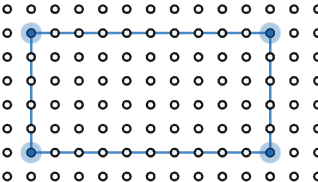Since the slope of a vertical line is undefined, one cannot use the slope formula to show that adjacent sides have negative reciprocal slopes ( and are not reciprocals!). To avoid this difficulty, one can instead use the fact that the diagonals of a rectangle are congruent, which can easily be checked using the distance formula.

## Perimeter and Area

Coordinate methods are not only useful for writing proofs, but can also be used to compute the perimeter and area of polygons. Computing the perimeter is straightforward: one simply uses the distance formula to compute the length of each side of the polygon, and then adds all of these lengths together.
Computing the area, as we will see, can be more subtle. Let us first consider the triangle below: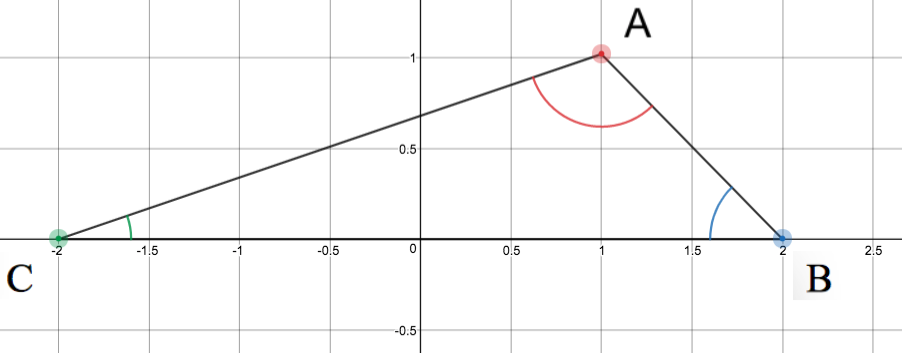The area is given by the familiar formula , where b is the base of the triangle and h is the height. Taking the base to be the segment, the height is the length of the altitude connecting vertex A to. Using the distance formula, it is easy to see thatand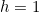, so the area of the triangle is square units.

This example was easy, but suppose you are instead given a triangle which is “tilted” in the coordinate plane, such as the one below:Taking againto be the base of the triangle, it is still straightforward to compute its length using the distance formula:

units.

However, the height is now much harder to compute! The altitude connecting vertexto the opposite side must intersectat a right angle. To find this segment, we can first find the equation of the line containing, which has the form. Using the slope formula, we find

.

Using the fact that the line must pass through point, the y-intercept,, is calculated by plugging in the coordinates of:

.

The equation of the line containingis therefore given by

.

We can now construct the line perpendicular to this line and passing through point. To be perpendicular to, the slope must be the negative reciprocal of the first, so this line has the form. We now solve foras before by plugging in the coordinates of:

.

The equation of the line containing the altitude throughis therefore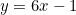. We now need to find the coordinates of the pointwhere these two lines intersect by solving the system of linear equations

By substituting the second into the first, we find the solution

.

Finally, we can use the distance formula to compute the height of the triangle:

The area of the triangle is thereforesquare units.

Fortunately, there are other ways to compute the area of the triangle which do not involve so much work. There is actually an alternative formula for the area of a triangle which avoids computing the height altogether. This is known as Heron’s formula. Denoting the side lengths of a triangle by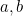and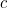. We first compute the semiperimeter,, of the triangle, which is simply half the perimeter:

.

Heron’s formula then states that the area is given by

.

(Note by the symmetry of this expression that it does not matter which side is labeledor.) This formula is obtained by noting that an altitude of a triangle partitions it into two right triangles. Applying the Pythagorean theorem to each of these smaller triangles, we can eliminatefrom the area formula, and, after some factoring, we arrive at Heron’s formula.

Returning to our example, using the distance formula we obtain

Plugging in to Heron’s formula (and a calculator!), we obtainsquare units.

By using Heron’s formula, we arrive at the answer with far less work than the previous method. Moreover, since Heron’s formula only uses the distance formula, it is straightforward to use for any triangle in the plane, regardless of its orientation.

We will now show another method which will allow us to compute the area with even less work. The trick is to draw a rectangle containing the triangle which passes through all of its vertices: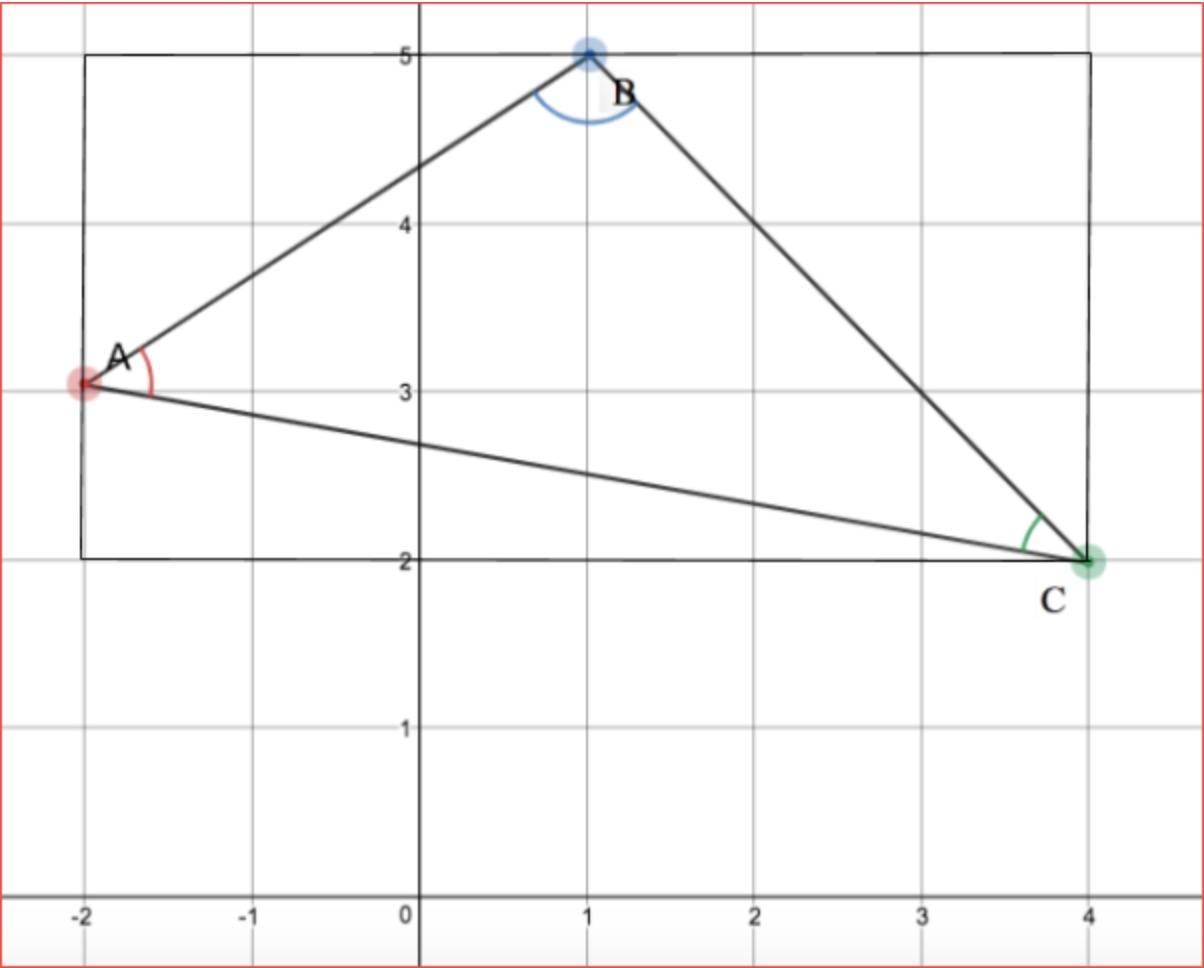We now see that the area of the triangle can be obtained by computing the area of this rectangle and subtracting the areas of the 3 right triangles at the corners:

square units.

These methods of computing areas can be extended to any polygon: since any polygon can be partitioned into triangles, we can use Heron’s formula repeatedly to compute the area of each triangle, and then add them all together. Alternatively, we can enclose the polygon in a rectangle and compute its area, subtracting off the areas of some number of right triangles. While the latter method is usually the easiest, it is not always guaranteed to work. For instance, consider the parallelogram below:In this case, enclosing the parallelogram in a rectangle does not create right triangles, but instead two concave quadrilaterals!

These examples illustrate the utility of a variety of different computational techniques, as there is no one method which is ideal for all situations. While the student must spend considerable time and effort mastering classical methods and the art of two column proofs, for many problems coordinate methods provide a powerful technique to quickly come to the same conclusion by straightforward, concrete calculations.

Be sure to give us a shout if you have any questions!